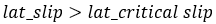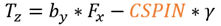# Force Calculation

All of the force calculations are done in the ISO coordinate system.

## Vertical Force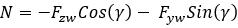Where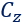is the vertical stiffness of the tire. The lateral deflection is ignored in the calculation.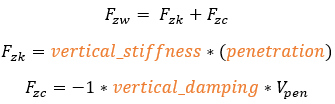Where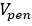is the tire penetration velocity.

The vertical force can only be positive: in the case of a lift off condition of the tire from the ground, the resulting negative reaction force is set to 0.

If a [DEFLECTION_LOAD_CURVE] block is present in tire property file, then the data inside the block is used to calculate the vertical force. In this case the VERTICAL_STIFFNESS value is ignored. The data in DEFLECTION_LOAD_CURVE should be monotonically increasing. An example of such table is given below: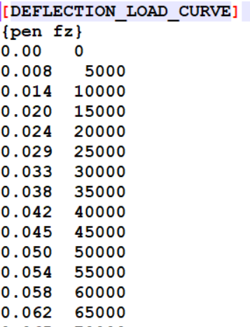## Friction Coefficient

The friction coefficient can be calculated in three ways:
1. Interpolating the Umax and Umin value using Comprehensive slip.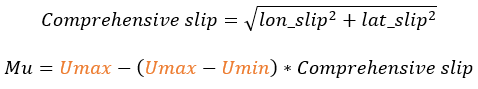2. Interpolating Mu using Vertical Force from a table.
3. Interpolating Mu using Slip from a table.

Option 1 is the default option. Option 2 or 3 can be used when you have a table representing Mu versus Vertical Force or Mu versus Slip as shown below:

In such a case, the parameter Mu must be set accordingly to the name of the block containing this table in the [parameter] block.

The table data must be provided within a block in the property file as shown below:

The name of the block MU_FZ or MU_SLIP must be entered between brackets defining the Mu parameter block, as in the figure above [MU_FZ] or [MU_SLIP]. There are three values to be provided in this block:
 INDEPENDENT_VARIABLE The friction coefficient is a function of this variable. There are two options: VERTICAL_FORCE and SLIP. DATA The sub table within this block containing the table data. INTERPOLATION Scheme used for interpolating the curve. There are four options: LINEAR, CUBIC, AKIMA, QUINTIC. Default = AKIMA.

To continue supporting previous versions, if the block [MU_SLIP_CURVE] is specified, it will be used without setting the Mu parameter block (MU_FZ or MU_SLIP). In such a case, the friction coefficient is calculated by interpolating the data in the table of the block [MU_SLIP_CURVE].

The data in [MU_SLIP] curve should be monotonically increasing. An example table is shown below: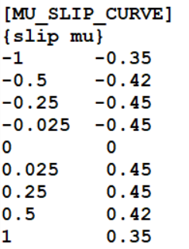## Longitudinal Force

The calculation of the longitudinal force (Fx) depends on the longitudinal slip condition of the tire. Typically, there are two distinct slip states: an elastic deformation state (where the longitudinal slip is less that a critical slip value) and a pure sliding state (where the longitudinal slip exceeds the critical slip value).

The critical longitudinal slip is calculated below: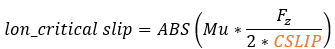In case of pure elastic deformation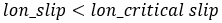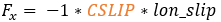In case of pure sliding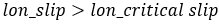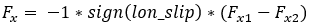Where: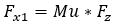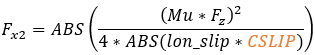## Lateral Force

Similar to the critical slip for longitudinal force, a critical lateral slip factor is calculated. It determines the boundary between pure elastic deformation conditions and pure sliding condition of the tire.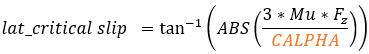In case of elastic deformation state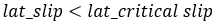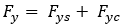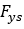and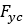are the lateral forces due to later slip and camber respectively.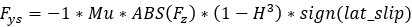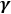is the camber angle, when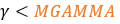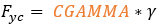And if>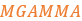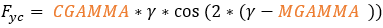Where: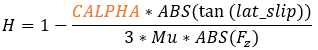In case of sliding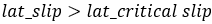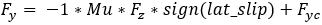## Overturning Moment

An overturning moment is caused by the shift of the contact point (at which the vertical force is applied) in the lateral direction.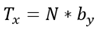## Rolling Resistance Moment

In the FIALA tire model the rolling resistance moment is purely a function of the normal force Fz: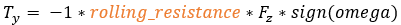Where omega is angular velocity of tire about spin axis.

## Aligning Torque

The same critical lateral slip used in the Fy calculation is also used in determining the condition of the tire.

In case of elastic deformation state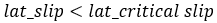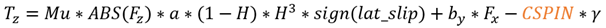Where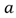is half of the contact patch length. The scaling factor here is half of the contact patch length, however in a FIALA model; the tire width is used as a scaling factor.

In case of sliding